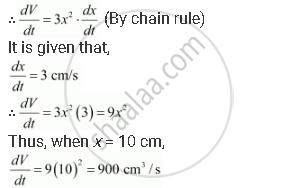# An Edge of a Variable Cube is Increasing at the Rate of 3 Cm/S. How Fast is the Volume of the Cube Increasing When the Edge is 10 Cm Long? - Mathematics

An edge of a variable cube is increasing at the rate of 3 cm/s. How fast is the volume of the cube increasing when the edge is 10 cm long?

#### Solution

Let x be the length of a side and V be the volume of the cube. Then,

V = x3.Hence, the volume of the cube is increasing at the rate of 900 cm3/s when the edge is 10 cm long.

Concept: Rate of Change of Bodies or Quantities
Is there an error in this question or solution?
Chapter 6: Application of Derivatives - Exercise 6.1 [Page 197]

#### APPEARS IN

NCERT Class 12 Maths
Chapter 6 Application of Derivatives
Exercise 6.1 | Q 4 | Page 197

Share